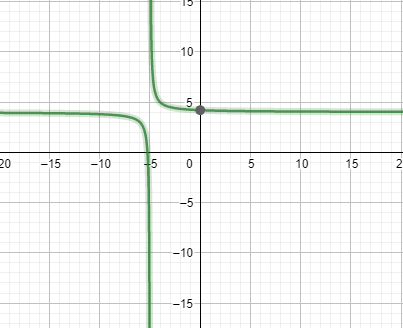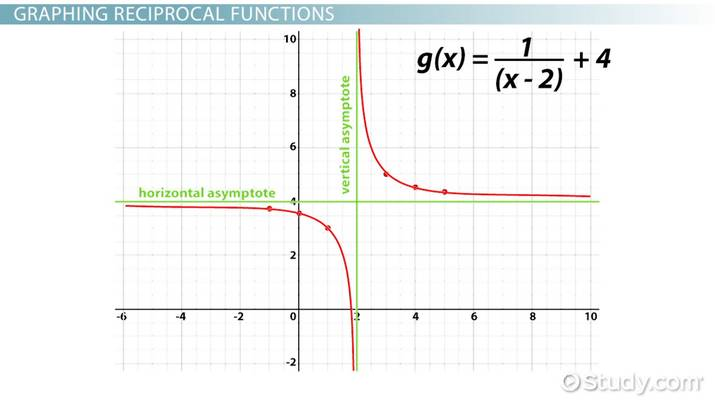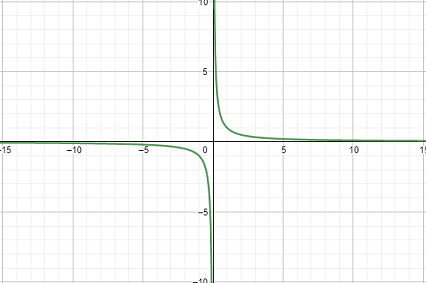# How to Use a Reciprocal Function

Laura Foist, Laura Pennington
• Author
Laura Foist

Laura has a Masters of Science in Food Science and Human Nutrition and has taught college Science.

• Instructor
Laura Pennington

Laura received her Master's degree in Pure Mathematics from Michigan State University, and her Bachelor's degree in Mathematics from Grand Valley State University. She has 20 years of experience teaching collegiate mathematics at various institutions.

What is a reciprocal function? View a reciprocal function graph and its traits, learn how to graph reciprocal functions, and see reciprocal function examples. Updated: 07/30/2021

Show

## What is a Reciprocal Function

A reciprocal function is the mathematical inverse of a function. In math, reciprocal simply means one divided by a number. So a reciprocal function is one divided by the function.

• The reciprocal of {eq}5 {/eq} is {eq}\frac{1}{5} {/eq}
• The reciprocal of the function {eq}x+5 {/eq} is {eq}\frac{1}{x + 5} {/eq}

The reciprocal function is the multiplicative inverse of the function. In other words, it is the function turned up-side down. Any function can be thought of as a fraction:

• {eq}x+5 {/eq} as a fraction is {eq}\frac{x+5}{1} {/eq}

Flipping the fraction upside down (making the denominator the numerator and the numerator the denominator) gives us the reciprocal function:

• {eq}\frac{1}{x+5} {/eq}

### How to Find a Reciprocal Function

Finding a reciprocal function is often simply a matter of flipping the fraction upside down. However, the difficulty lies in getting the function into the standard reciprocal function form. The standard reciprocal function form is:

{eq}\frac{a}{x-h} + k {/eq}

Where:

• a is a constant number
• x is the x-variable in the f(x)
• h is the vertical asymptote
• k is the horizontal asymptote

Take a look at the function:

• {eq}f(x)=x+7 {/eq}

The inverse of this function is:

• {eq}\frac{1}{x+7} {/eq}

This is almost in the standard form for reciprocal functions:

• a = 1
• x = x
• h = -7
• k = ?

Since there are no other terms in this equation, it is implied that "k" is 0:

• {eq}\frac{1}{x+7} + 0 {/eq}

### Finding the Standard Reciprocal Form

Some functions are a little harder to find in the standard reciprocal form. For example, the function:

• {eq}\frac{x+5}{4x+21} {/eq}

The inverse function is:

{eq}\frac{4x+21}{x+5} {/eq}

This function does not fit the standard reciprocal function form. There are a couple of methods that can be used to find the standard reciprocal function form:

• Create a table of x and y values for the function and identify the vertical and horizontal asymptotes
• Graph the function (using a graphing tool or by hand) and identify the vertical and horizontal asymptotes

First, create a table of x and y values:

x value y value
-15 3.9
-10 3.8
-5 Not real number
0 4.2
5 4.1

The vertical asymptote is the x-value when the denominator equals zero; this is illustrated when the y-value is a non-real number (because it doesn't exist). Thus, in this equation, the vertical asymptote is -5. The horizontal asymptote is the number that is being approached in both the positive and negative directions. In this case, 4 is being approached, so the horizontal asymptote is 4.

This means that the reciprocal function in standard form has the following numbers:

• a = 1
• x = x
• h = -5
• k = 4

Making the formula:

• {eq}\frac{1}{x+5} + 4 {/eq}

The other method is looking at a graph of the function:This graph shows the vertical asymptote at 4 and the horizontal asymptote at -5, giving the same equation as above. The next section discusses what happens if 'a' is not equal to 1 and x is not equal to x.An error occurred trying to load this video.

Try refreshing the page, or contact customer support.

Coming up next: How to Add, Subtract, Multiply and Divide Functions

### You're on a roll. Keep up the good work!

Replay
Your next lesson will play in 10 seconds
• 0:04 What is a Reciprocal Function?
• 1:36 Reciprocal Function…
• 3:14 Graphing Reciprocal Functions
• 4:24 Lesson Summary
Save Save

Want to watch this again later?

Timeline
Autoplay
Autoplay
Speed Speed

## Reciprocal Function Graph

The simplest reciprocal function is {eq}\frac{1}{x} {/eq}

In this case:

• a=1
• x=x
• h=0
• k=0

The graph of this function is:All reciprocal functions have a graph with two sections (called branches), where both curves go off towards infinity towards the vertical and horizontal asymptotes. In this simple reciprocal function, both the horizontal and vertical asymptotes are 0, so the curves are centered around the x and y axis. Increasing the horizontal asymptote will move the entire graph up. Decreasing the horizontal asymptote will move the entire graph down. Increasing the vertical asymptote will move the entire graph to the right while decreasing the vertical asymptote will move the entire graph to the left.

Increasing the value of 'a' will cause the curve to get less steep:

To unlock this lesson you must be a Study.com Member.

### Register to view this lesson

Are you a student or a teacher?